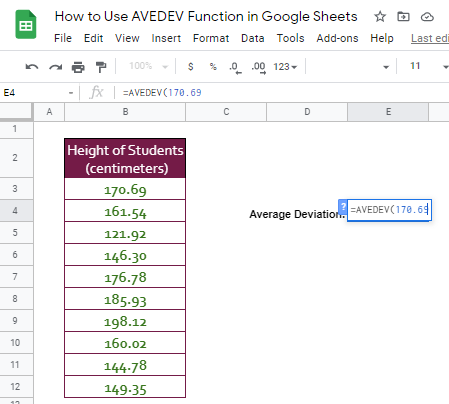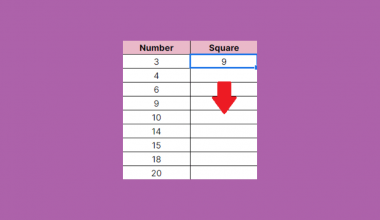# How to Use AVEDEV Function in Google Sheets

The AVEDEV Function in Google Sheets is useful if you want to find out the average of the absolute value of deviations from the mean for a given set of values.

Meaning, it is used to measure how far, on average, all given values are from the middle. This function is commonly used by professionals in accounting, investing, and economics.

The rules for using AVEDEV function in Google Sheets are as follows:

• Arguments can be numbers, cell range, arrays, or references that contain numbers.
• The function ignores empty cells, and cells that contain text or logical values. However, cells with the value zero are included
• If you use text, blank cells, error or Boolean values directly as arguments in the formula, AVEDEV will return an error.
• Likewise, if all your argument values are strings, you are going to get an error.

Let’s take an example.

Anne, a professor in junior high, wants to know how her students’ performed after a 150-item test. Her data is shown below:Anne here averaged the scores. However, it can only tell a bit of information. The average gives you the central value of data, but does not tell you about the spread. It could mean that some of her students could have gotten almost perfect scores, which skewed the average. But, her other students may have performed poorly.

She used the AVEDEV function to get the average deviation of the given scores. See the results below:The average deviation tells that, on average, her students’ scores are 25 points from the mean, or the mean average.

Let’s have another real-life sample!

Janine is planning to invest in ABC company by buying shares. The company reported last year that the average return year on year for the last 3 years was at 19%. Looks promising, right?

What the report isn’t possibly telling her is that, in the first year, the company yielded 70% return, -9% and -5% in the second and third year, respectively.

Since she could analyze further by calculating the average yield and the average deviation year on year for the past 3 years, Janine would now reconsider other options.

Watch out for a more advanced tutorial and examples on how you can use AVEDEV function in the coming weeks. Be sure to subscribe to be notified.

Awesome! Let’s begin getting to know more about our AVEDEV function in Google Sheets.

## The Anatomy of the AVEDEV Function

So the syntax (the way we write) the AVEDEV function is as follows:

`=AVEDEV(value1, [value2,value3,...])`

Let’s dissect this thing and understand what each of these terms means:

• = the equal sign is just how we start any function in Google Sheets. It is how Google Sheets understand that we are asking it to either do a computation or use a function.
• AVEDEV() this is our AVEDEV function. AVEDEV will take the values and compute for the average deviation.
• value1 is the first value or reference to the range of the dataset.
• value2,value3,… [optional] are the additional values or references to ranges that contain values we need to include in the dataset.

## A Real Example of Using AVEDEV Function

Take a look at the example data set below to see how AVEDEV function is used in Google Sheets.As you can see, I used different functions and formulas to be able to come up with the average deviation of the given score set.

Basically, for you to be able to get the average deviation, first, you’ll need to get the mean or the average of the given values (See cell B16). After which, you need to subtract each value from the average value (See Column C for the result and Column D for the formula used in Column C). There should be no negative results. Hence, the absolute function would help you achieve that (See Column E for the result and Column F for the function used in Column E). Finally, average the absolute differences yielded (See cell E16).

In the given sample above, it means that, on average, the students’ scores are 35 points from the mean, which is 104 points.

Now, please see the table below and how AVEDEV function is used to obtain the same result as above:See how a simple function makes someone’s job easier? That’s how useful this function is to financial professionals.

You may make a copy of the spreadsheet using the link I have attached below.

## How to Use AVEDEV Function in Google Sheets

1. Simply click on any cell to make it the active cell. For this guide, I will be selecting E4, where I want to show my result.1. Next, type the equal sign ‘=‘ to begin the function and then followed by the name of the function, which is our ‘avedev‘ (or ‘AVEDEV‘, not case sensitive).

1. Type open parenthesis ‘(‘ or simply hit Tab key to let you use that function.1. Now the fun part! Let’s give our function the argument, which is the list of given values. You may pass a constant data by typing each of the given values after the parenthesis.1. To let the Google sheet know that we’re done typing our first argument, we should now type in the delimiter or the character that separates each argument on a function. In this case, type comma,’ followed by the next argument, 161.54.1. Separated by the delimiter, the rest of the values should follow.1. Finally, just hit your Enter or Tab key. The cell E4 is now showing you the result or the return value of the AVEDEV function.1. Notice that, in our example, we provided the exact values, constant, as the arguments of our AVEDEV function. Alternatively, these constants can be variable or simply cell addresses or ranges of your data.

1. In cell E7, follow steps 1-7. Only this time, instead of the actual given values, we will use the cell range where your set of values is located. In this case, the range B3:B12.1. Check and compare the results in cells E4 and E7. They should be the same.That’s pretty much it.  You can now use the AVEDEV function in Google Sheets together with the other numerous Google Sheets formulas to create even more powerful formulas that can make your life much easier.Our goal this year is to create lots of rich, bite-sized tutorials for Google Sheets users like you. If you liked this one, you'll love what we are working on! Readers receive ✨ early access ✨ to new content.

##### You May Also Like## How to Use ISEMAIL Function in Google Sheets

The ISEMAIL function in Google Sheets is used to determine if a value is a valid e-mail address.…## How to Use the ACCRINTM Function in Google Sheets

The ACCRINTM function in Google Sheets is designed specifically to calculate the interest that is paid at maturity…## How To Use ISREF Function in Google Sheets

The ISREF function in Google Sheets is useful when you need to check if a certain value is…## How to Use the XIRR Function in Google Sheets

The XIRR function in Google Sheets is used to calculate the internal rate of return on investment based…